• Call Now

1800-102-2727•

# Modulation and Demodulation

You might have used a device called “Modem” to use the internet in your computer system. This device is basically a “Modulator and Demodulator” device which is used to send digital information to a receiving device (like a computer or the internet line). A modem converts the digital signal into the signal which is compatible with the receiving device at the receiving modem side and then it is again converted into a digital signal for decoding the correct information. This whole process is known as modulation and demodulation which we will cover in this topic. A wireless modem is used to convert a digital signal into a radio signal and vice-versa.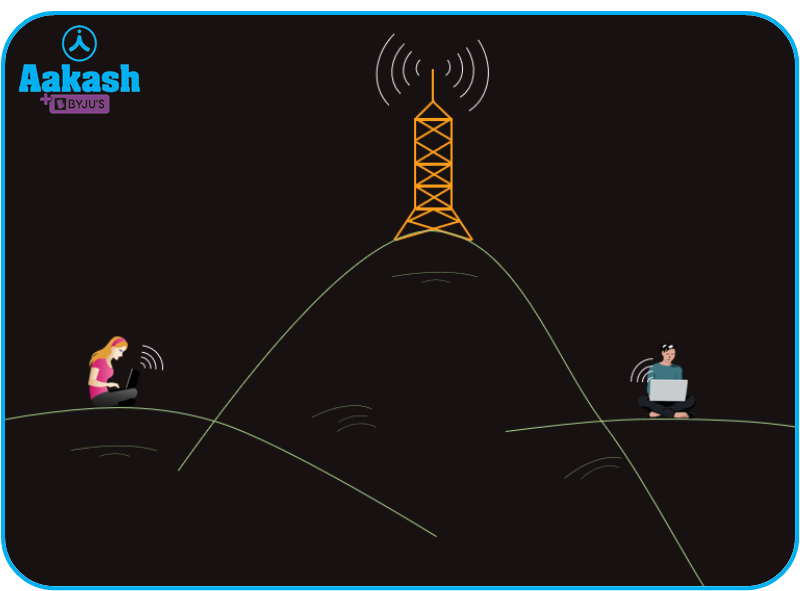Table of content

• Definition of message signal
• Carrier wave
• What is Modulation?
• Importance of modulation
• Types of modulation
• What is Demodulation?
• Working of modulation and demodulation
• Practice problems
• FAQs

## Definition of message signal

A Message signal is an electrical signal generated from the original information that is to be transmitted, using a transducer. This is also known as the baseband signal. Every information from a device needs to be converted into a form through which it can be transmitted from that device to another.

There are two types of message signals,

1. Analog signal: Analog signals are the signals in which the voltage and the current vary continuously with time. For example; sound, music, the sound produced by a tuning fork, etc.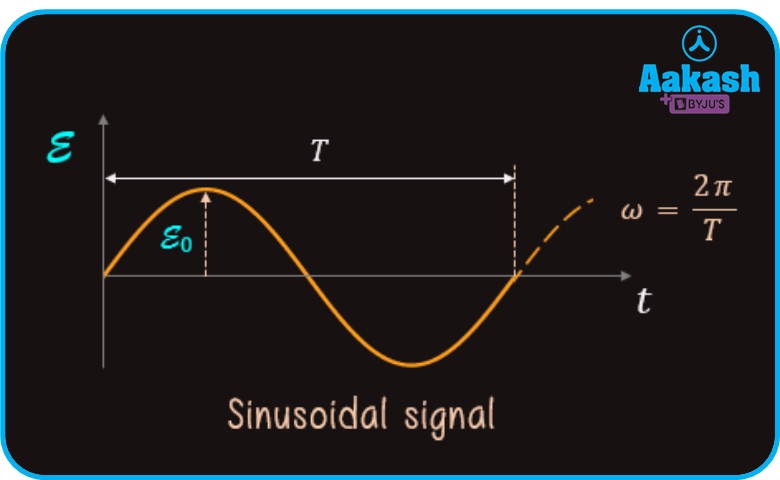1. Digital signals: In a digital signal, the voltage and current vary with time in the form of a pulse which is discontinuous in nature. The digital signal has only two values, high and low which is represented in binary format as 0 and 1. For example; output of a computer is a digital signal.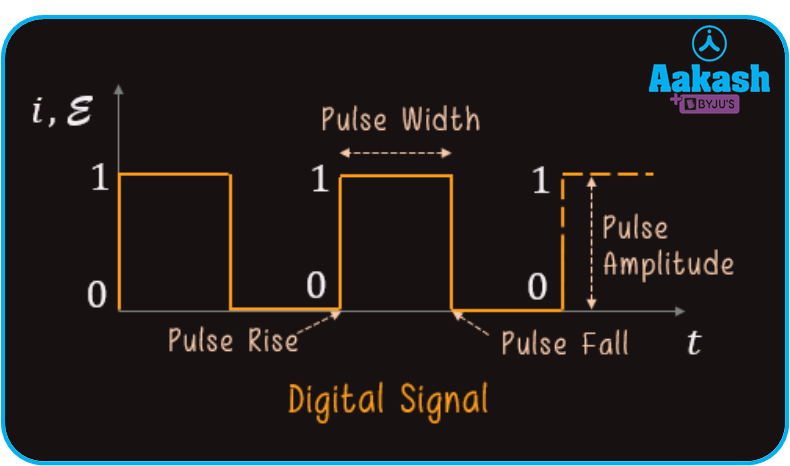## Carrier wave

Carrier wave is a high-frequency signal which is responsible for the transmission of a message signal to a receiver. A carrier signal is represented as,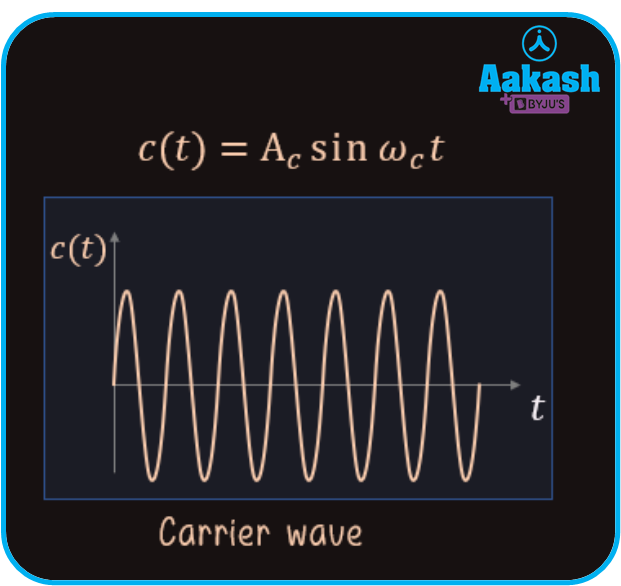There are two types of the carrier wave,

(i) Sinusoidal (continuous) wave

(ii) Discontinuous (pulsed) wave

## What is modulation?

Modulation is a technique to send certain information in the form of a signal superimposed over a high-frequency signal known as the carrier signal by transmission. The device based on this technique is known as the modulator. The message signal has a low frequency that can not be transmitted without the help of a carrier signal which is a high-frequency signal.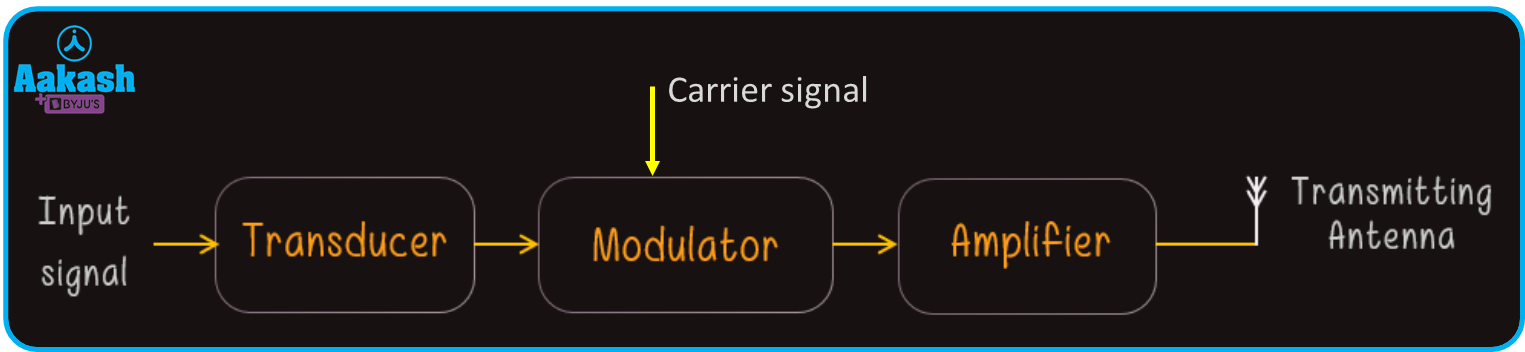## Importance of modulation

1. This step is needed before the transmission of a signal in order to avoid the mixing of signals generated from different transmitters, so that a good quality signal can be received at the receiving end.

2. Power radiated from the antenna: If the power radiated from the antenna is P, length of the antenna is l and the wavelength of the transmitting signal is , then

As seen from the above equation, the effective radiated power is inversely proportional to the wavelength of the transmitting wave for a given length of the antenna. Therefore for a good quality of transmission, the power radiated should be high i.e., the wavelength of the transmitted wave should be small or the frequency should be high. This is done by using a high-frequency carrier wave.

3. Height of the antenna: Modulation is one of the important ways to optimize the height of the antenna. In modulation, a carrier signal of very high frequency is used to transmit the actual low-frequency message signal.

If c is the speed of the wave, f is the signal frequency then, the wavelength corresponding to the message signal is given by,

$\lambda =\frac{c}{f}$

Now we know that, for a proper transmission of signal, the minimum height of the antenna is,

$L=\frac{\lambda }{4}$

If we want to transmit a message signal without modulation, the height of the antenna would be too high because the frequency of the message signal is very low in general. After the modulation, the frequency of the modulated wave is sufficiently increased so that the wavelength of the modulated signal is reduced. Hence, the minimum length of the antenna is also reduced.

## Types of modulation

Based on the nature of the carrier wave, there are two types of modulation,

1. Continuous wave modulation
2. Pulse wave modulation

These modulations are again divided into several parts based on the variations in amplitude, phase and frequency of the carrier signal and the information signal.

(a) Continuous wave modulation:

1. Amplitude modulation: If the modulation is done in such a way that there is a difference in amplitudes of the information signal and the carrier signal while keeping the frequency and the phase of the carrier signal and the modulated wave constant, then this type of modulation is amplitude modulation.

If c(t) represents the carrier wave and m(t) represents the message signal and their respective equations are, c(t)=Acct and m(t)=Ammt , where, Ac and Am are the peak amplitudes of carrier wave and message signal respectively. The waveforms of carrier wave and message signal are given below,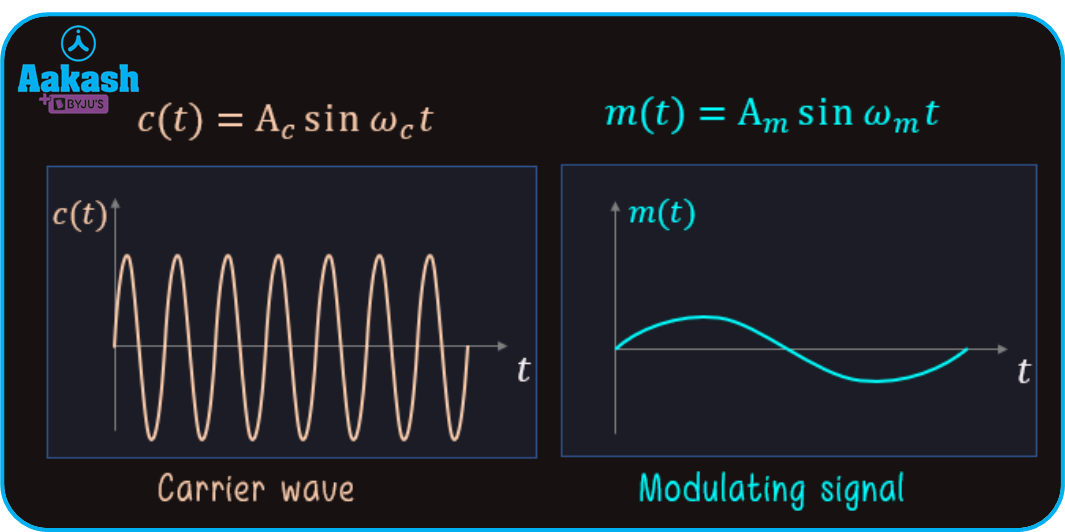Now during modulation, the carrier wave and the message signal are superimposed and the modulated wave is known as the amplitude modulated wave.

The equation of the AM wave is represented as,

${c}_{m}\left(t\right)={\left(A}_{c}+{A}_{m}sin{\omega }_{m}t\right)sin{\omega }_{c}t$

${c}_{m}\left(t\right)={A}_{c}sin{\omega }_{c}t+\frac{\mu {A}_{c}}{2}\left[cos\left({\omega }_{c}-{\omega }_{m}\right)-cos\left({\omega }_{c}+{\omega }_{m}\right)\right]$

Where, $\mu =\frac{{A}_{m}}{{A}_{c}}=$ modulation index

The waveform of the AM wave is depicted below,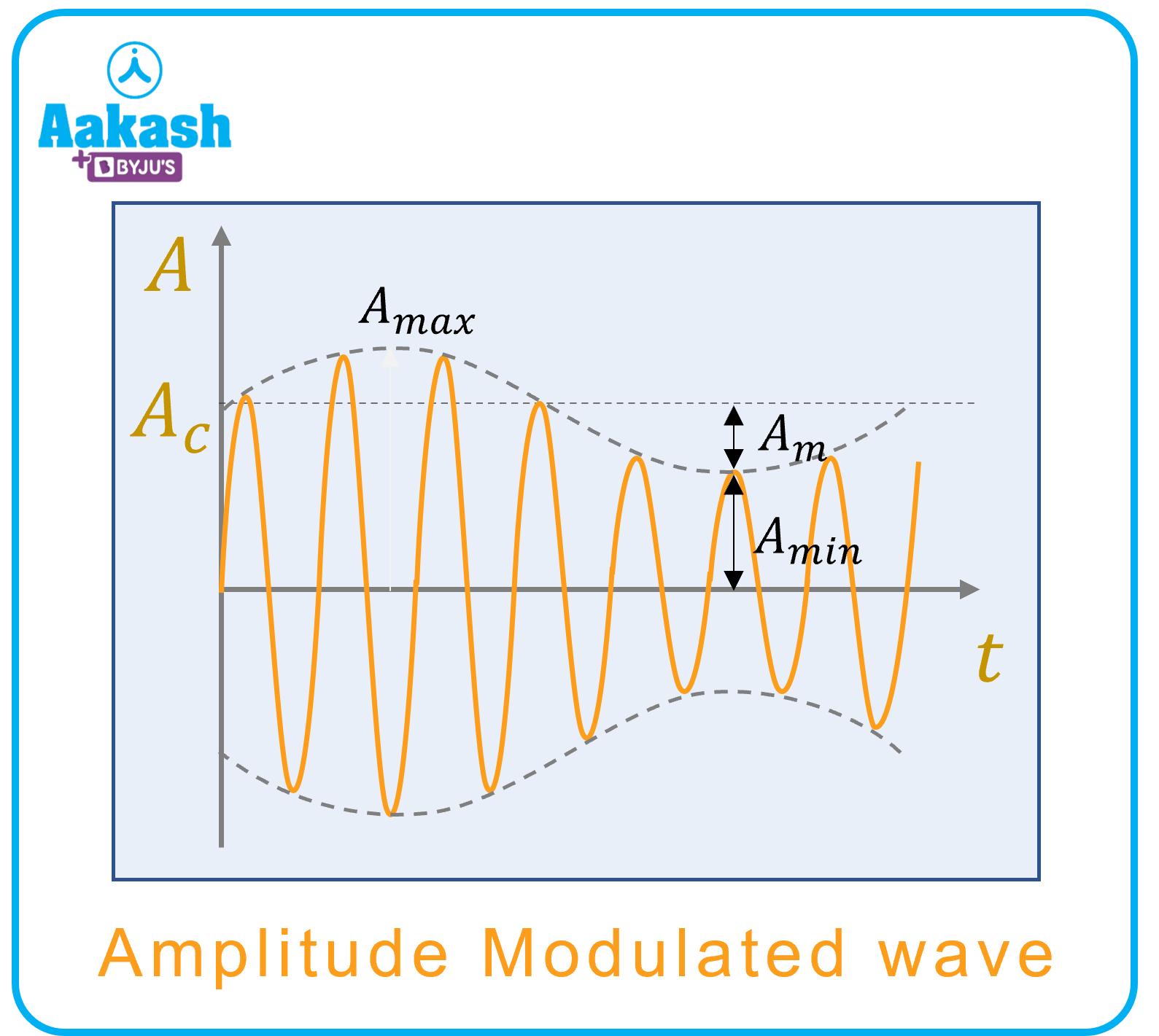As seen from the above figure, the maximum and minimum amplitudes of the AM wave is,

${A}_{max}={A}_{c}+{A}_{m}and{A}_{min}={A}_{c}-{A}_{m}$

If are the lower side frequency and upper side frequency

respectively, then

Bandwidth of an AM wave $={f}_{USB}-{f}_{LSB}$

Where,

Bandwidth of an AM wave $=\left({f}_{c}+{f}_{m}\right)-\left({f}_{c}-{f}_{m}\right)$

=2fm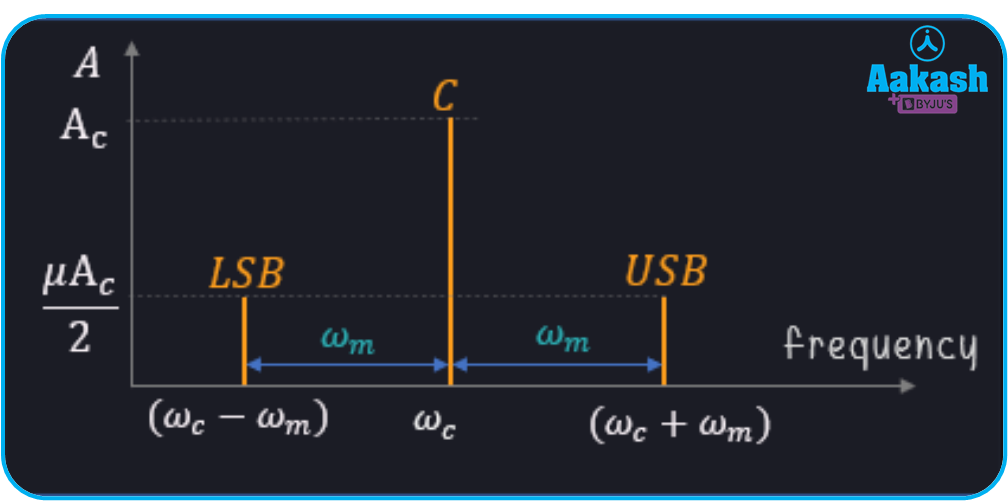Plot between amplitude (A) and for an AM wave

Total power in an amplitude modulated wave is, ${P}_{t}={P}_{c}+{P}_{USB}+{P}_{LSB}$

Where,

Pc= Power consumed by carrier wave

PUSB= Power consumed by upper sideband

PLSB= Power consumed by lower sideband

${P}_{t}={P}_{c}+\frac{{\mu }^{2}}{4}{P}_{c}+\frac{{\mu }^{2}}{4}{P}_{c}$

${P}_{t}={P}_{c}\left(1+\frac{{\mu }^{2}}{2}\right)$

Where, = modulation index of AM wave

1. Frequency modulation: If the modulation is done in such a way that there is a difference in frequencies of the information signal and the carrier signal by keeping amplitude and phase of the carrier signal and the modulated signal constant, then this type of modulation is frequency modulation.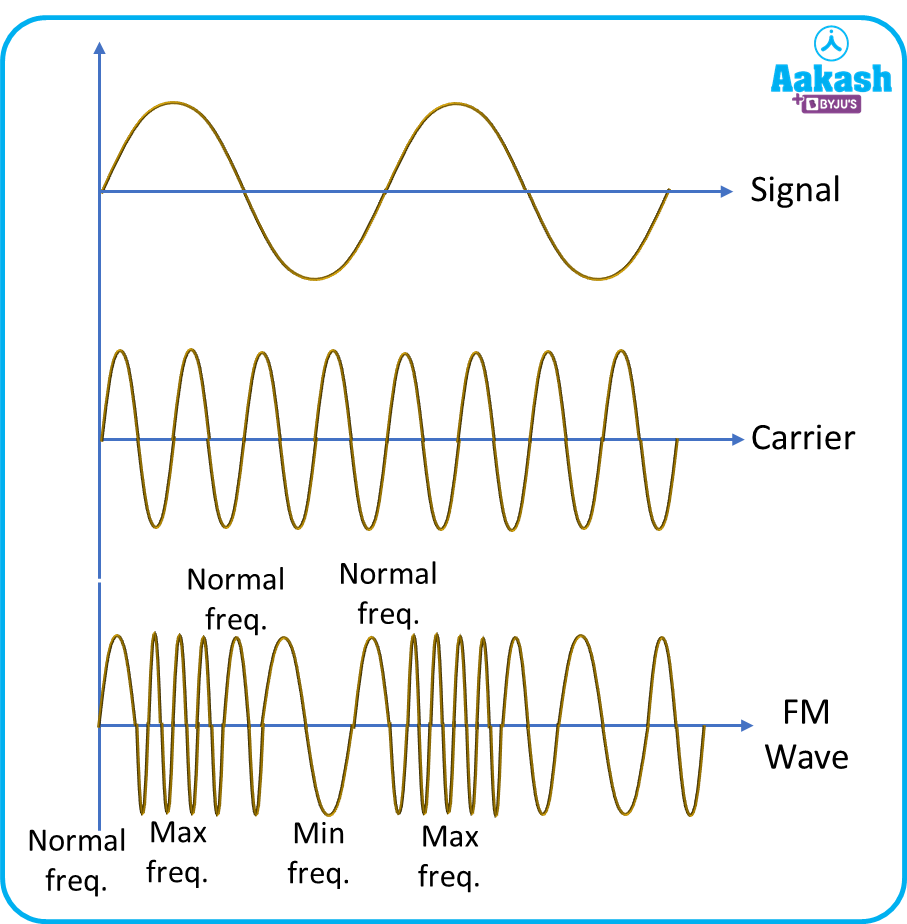The modulation index for the FM wave is,

1. Phase modulation: If the modulation is done in such a way that there is a difference in phase between the information signal and the carrier signal while keeping frequency and amplitude of the carrier signal and the modulated signal constant, then this type of modulation is frequency modulation.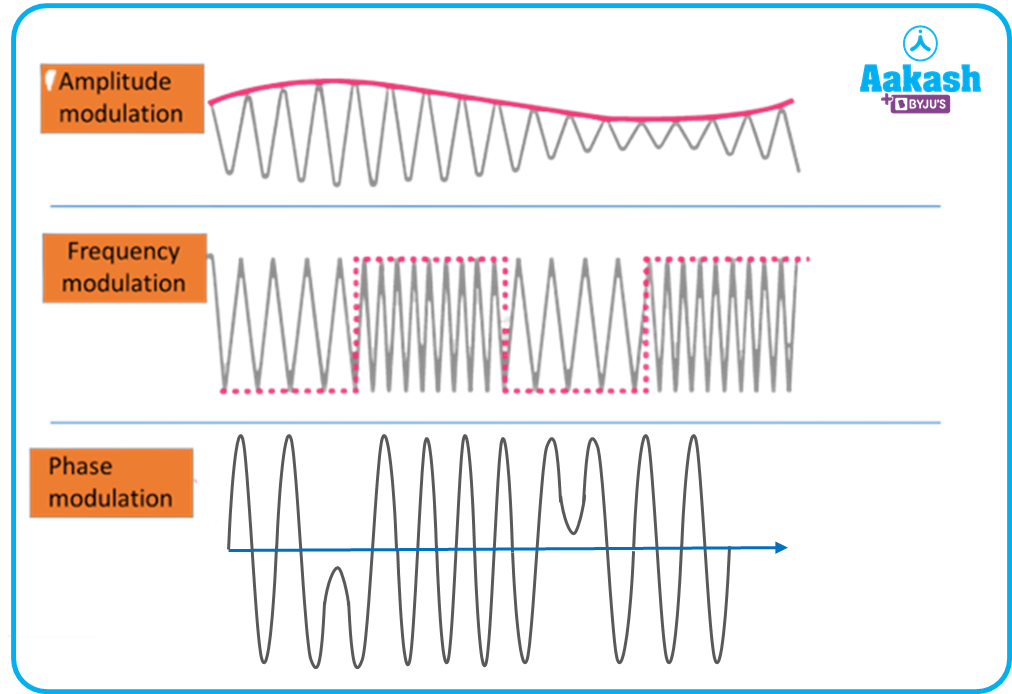Figure shows the AM waves, FM waves and PM waves

(b) Pulse wave modulation

1. Pulse amplitude modulation
2. Pulse width modulation
3. Pulse position modulation

## What is Demodulation

Demodulation is the process of filtering the message signal from the carrier signal at the receiver end when the modulated signal is received. It is an important step to get correct information from the message signal. After receiving the modulated signal at the receiver end, there is no use of the carrier signal. Hence, it has to be removed.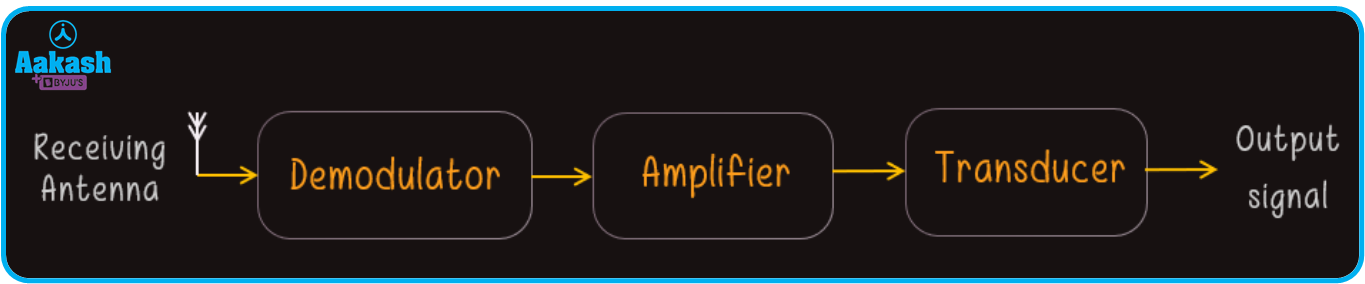## Working of modulation and demodulation

Let’s say information is to be sent to a certain place which is very far from it. Then the information will be converted into a certain form of signal which can be transmitted through the communication channel. After receiving the transmitted signal at the receiver end, it will be converted into its original form that will give the required information to the receiver.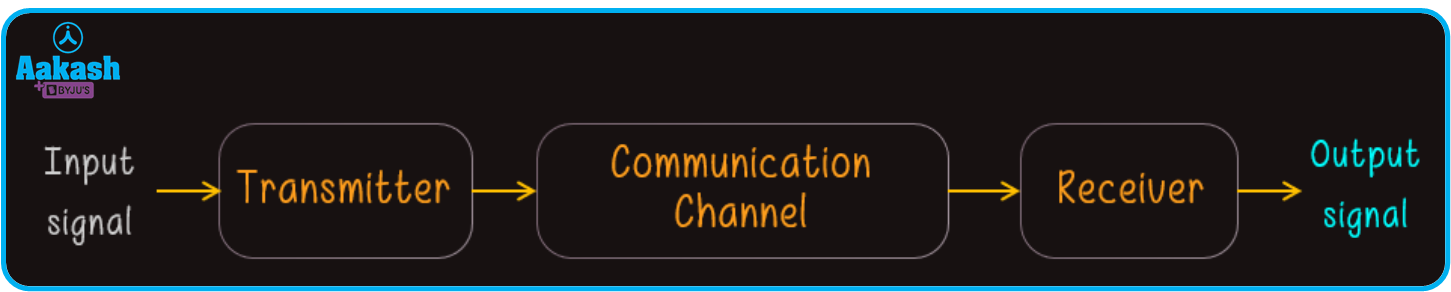In this process, the information is given to a transmitter as an input which will convert it into a signal that can be transmitted. This could be in the form of a radio signal or an electric signal.

In the transmitter, there are multiple parts, used to convert an input signal into a signal which can be transmitted via a communication channel. The parts and their workings are described below.Transducers: Transducers are devices that convert signals of various physical forms into electrical signals, and vice versa. If the transducer is used at the transmitting end, then it will convert the input signal into an electrical signal, known as the message signal.

Example: Microphone, touchpad of a mobile phone, photo-detectors.

Modulators: Then the modulator will modulate the message signal by superimposing a high-frequency carrier signal onto it since the message signal has a very low frequency. By modulating the message signal, it can be easily transmitted to the receiver.

Amplifier: The power of the modulated signal is boosted using a suitable amplifier.

Transmitting Antenna: After amplification of the modulated signal, it is radiated into space with the help of an antenna.

Now the radiated signal is sent to the receiver with the help of a proper communication channel. Some of the communication channels are depicted below.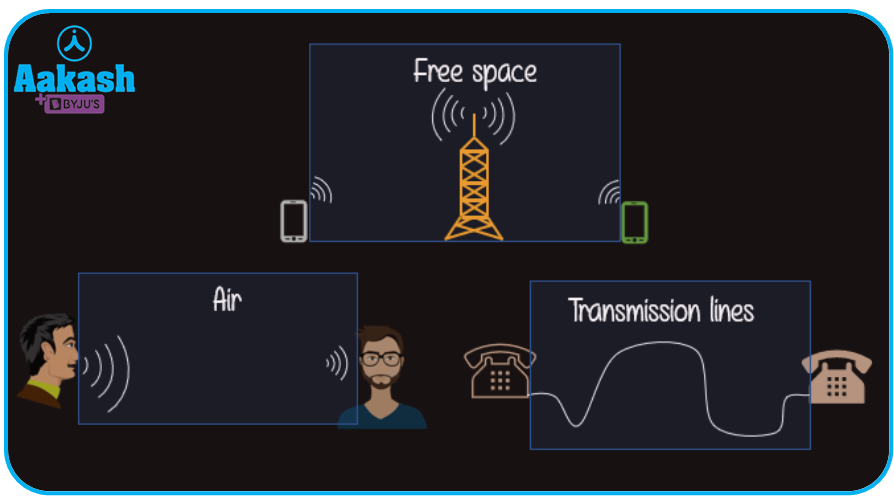After transmission through the communication channel, the signal is received by the receiver. The signal as received is to be converted into an appropriate type of signal. Similar to the transmitter, the receiver also has various parts for converting the electrical signal as explained below.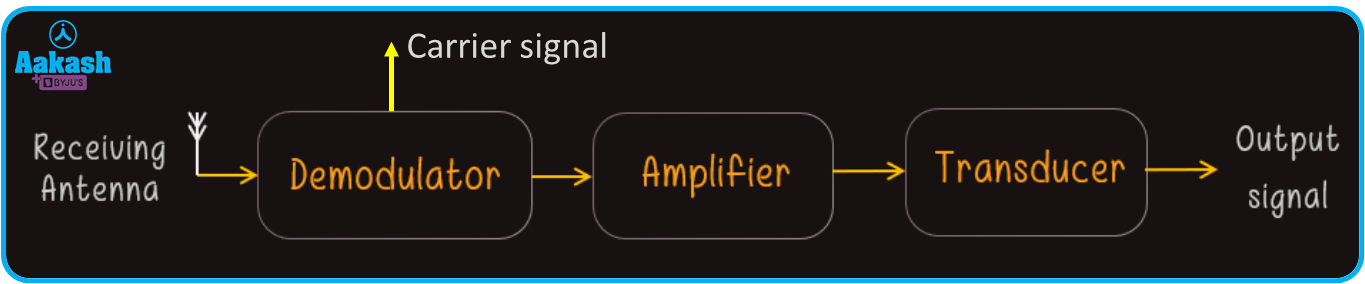Receiving antenna: The receiving antenna picks up the signal and sends it to the demodulator.

Demodulator: The demodulator then demodulates the message signal. It means that the demodulator separates the carrier signal and the message signal. Since after receiving the modulated signal, there is no use of the carrier signal and it has to be separated from the message signal.

Amplifier: The power of the message signal is boosted using a suitable amplifier.

Transducers: As we have discussed, transducers are devices that convert signals of various physical forms into suitable electrical signals and vice versa. Now, if the transducer is used at the receiving end, then it will convert the message signal into its original information signal.

## Practice problems

Q. A carrier signal having frequency of 2 MHz is being used to transmit a message signal then find the required length of the antenna for transmitting the signal.

Ans: Given, frequency of the carrier signal f=2 MHz=2106 Hz

Now, the corresponding wavelength will be,

Where, c= speed of wave =3108 m/s

Then from equation (i),

Now we know, for a good transmission, the minimum length of the antenna is given by,

$L=\frac{\lambda }{4}=\frac{150}{4}$

L=37.5 m

Hence, the length of the antenna should be 37.5 m, corresponding to the carrier signal having frequency of 2 MHz.

Q. A message signal of frequency 1 kHz is superimposed on a carrier signal of frequency 100 KHz. The modulation is done by using frequency modulation technique and the maximum frequency deviation in the modulated signal is 10 kHz. Find the modulation index for the FM wave.

Ans: Given, frequency of message signal fm=1 kHz

Frequency of carrier signal fc=100 kHz

And the maximum frequency deviation of modulated signal f=10 kHz

Then the modulation index of the FM wave is given by,

$\mu =\frac{\mathrm{\Delta f}}{{f}_{m}}$

$\mu =\frac{10}{1}=10$

Q. A broadcasting channel radiates 5 kW power with a modulation index 60%. Calculate the carrier power consumed by the carrier signal.

Ans: Given, The radiated power or total power, Pt=5 kW=5103 W

Modulation index, =60%=0.6

The total power is,

${P}_{t}={P}_{c}\left(1+\frac{{\mu }^{2}}{2}\right)$

Then the carrier power will be,

Q. The maximum and minimum amplitudes of a amplitude modulated wave are 30 V and 15 V. Calculate the modulation index.

Ans: Given, max. amplitude of message signal Amax=30 V

min. amplitude of carrier signal Amin=15 V

Then the modulation index is given by,

$\mu =\frac{30-15}{30+15}=\frac{15}{45}$

$\mu =0.33=33.33%$

## FAQs

Q. Define the measuring wave.
A.
The wave which contains the information and has a very low frequency is known as a measuring wave. It is also known as the message wave. For the transmission of this measuring wave, a high-frequency carrier wave is used.

Q. Which modulation is better, frequency or amplitude?
A.
In the frequency modulation, the amplitude of the FM wave remains constant, which makes it easier to remove the low frequency wave from the modulated signal. But this is difficult to do in amplitude modulation therefore frequency modulation is better than amplitude modulation.

Q. In a communication system at what stages modulation and demodulation is done?
A.
Since modulation is the process of providing enough strength to the message signal by superimposing it with a carrier signal, then it has to be done on the transmitter side and is done by a modulator. Demodulation is the process of separating the carrier signal and recovering the message signal from the modulated signal. So, it has to be done on the receiver end and is done by a demodulator.

Q. Define over-modulation?
A.
When the amplitude of a message signal becomes greater than a carrier signal during modulation then it is known as overmodulation. It is avoided by keeping the amplitude of the carrier signal greater than the message signal.Talk to our expert
Resend OTP Timer =
By submitting up, I agree to receive all the Whatsapp communication on my registered number and Aakash terms and conditions and privacy policy